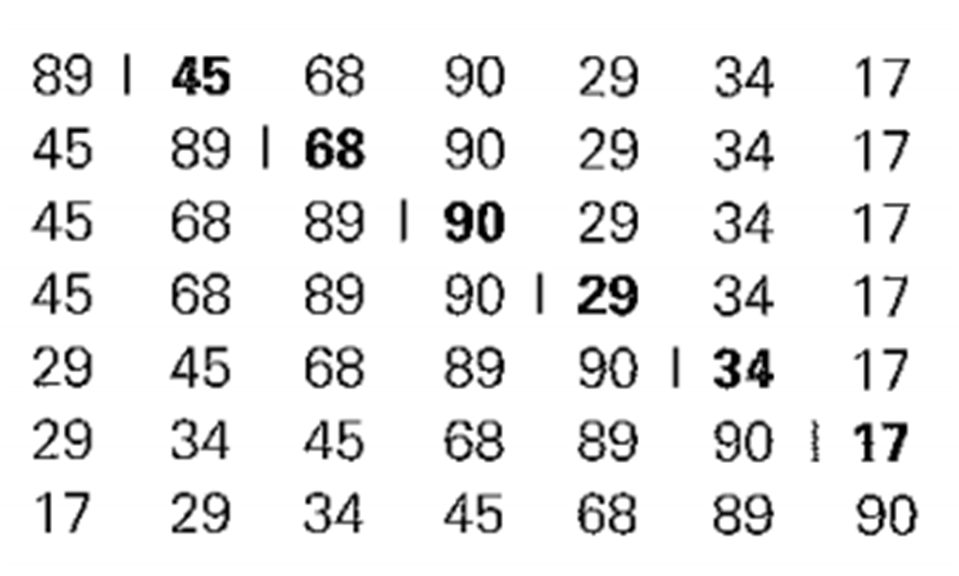## INSERTION SORT IN C

In Insertion Sort we select a key i.e an element one by one from any given list of element ( array ) and then we insert it in its appropriate position. We can either scan the list from left to right or right to left to find an appropriate position. But usually we scan list from right to left because it is better in case of sorted and almost sorted arrays. Insertion sort is an efficient algorithm for sorting small number of elements. Though Insertion Sort is based on recursive idea, it is more efficient to implement this algorithm by bottom up approach i.e iteratively.

Consider the elements to be sorted by insertion sort are : 89, 45, 68, 90, 29, 34, 17

As shown in Figure below, starting with A and ending with A[n - 1], A[i] is inserted in its appropriate place among the first i elements of the array that have been already sorted (but, unlike selection sort, the elements are generally not in their final positions).Insertion Sort In C : Showing how elements are sorted
The above figure shows how elements 89, 45, 68, 90, 29, 34, 17  are sorted by insertion sort. A vertical bar separates the sorted part of the array from the remaining elements; the element being inserted is in bold.

Note : Refer to algorithm for better understanding.

## INSERTION SORT ALGORITHM

```InsertionSort(int a[ ], int n)

for i=1 to n-1
key = a[i]
j = i-1```
```
while j>=0 and key < a[j]
a[j+1]=a[j];
j=j-1;

a[j+1] =  key
```

Note : The operation of the algorithm can be understood with the help of above figure.

## TIME COMPLEXITY OF INSERTION SORT

### Time Complexity Of Insertion Sort - Best Case

• The best case input in an array is such that the array is already sorted.
• In this case insertion sort has linear running time i.e O(n)

### Time Complexity Of Insertion Sort -Worst Case

• The worst case input in an array is such that the array is sorted in reverse order.
• In this case insertion sort has quadratic running time i.e O(n2

### Time Complexity Of Insertion Sort -Average Case

• Input in this case is a random input.
• In this case also insertion sort has quadratic running time i.e O(n2)
• Insertion Sort is very efficient in sorting very small arrays.
• Its impractical to sort very large arrays using insertion sort due to its time complexity of O(n2)

## INSERTION SORT PROGRAM IN C

```// Insertion sort program in c

#include<stdio.h>

void InsertionSort(int a[],int n) ;

int main()
{
int a,i,n;
printf("Enter number of elements in array : ");
scanf("%d",&n);

// Inputting elements
for(i=0;i<n;i++)
{
printf("Enter number %d: ",i+1);
scanf("%d",&a[i]);
}

// Displaying Elements before insertion sort
printf("Items in the array are : ");
for(i=0;i<n;i++)
{
printf("%d ",a[i]);
}

//Applying insertion sort
InsertionSort(a,n);

// Displaying elements after insertion sort
printf("\nElements after insertion sort : ");
for(i=0;i<n;i++)
{
printf("%d ",a[i]);
}
return 0;
}

void InsertionSort(int a[],int n)
{
int i,key,j;

for(i=1;i<n;i++)
{
key=a[i];
j=i-1; ```
```
```
```  // Finding appropriate position to insert key
while((key<a[j])&&(j>=0))
{
a[j+1]=a[j];
j=j-1;
}
```
```  // Inserting key
a[j+1]=key;
}
}

```